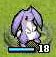# 星之一角

What have you found for these years?

## 2009-03-15

### 印星星 (2)

...頭一次碰到有人來踢館，還溝通不能，真無趣。

Camlp4ListComprehension 似乎是可以用，但是...

let ... in let ... in let ... in ......... orz

print 也是一堆 print_xxx ... 連要測試都很困難。

parameter 跟 fold_left 居然是反過來的 @@

ocaml byebye...

`(* Star.ml *)(*#load "dynlink.cma";;#load "camlp4/camlp4o.cma";;#load "camlp4/Camlp4Parsers/Camlp4ListComprehension.cmo";;*)let rec range i j = if i > j then [] else i :: range (i+1) j;;let star max =  let mid   = max / 2 + 1 in  let spaces i  = List.map (fun x -> " ") (range 0 (abs (mid-i-1) - 1)) in  let row n     = List.map string_of_int                    (range mid (( max+mid-2 * abs (mid-n-1) ) - 1)) in  let rectangle = List.map (fun i -> List.append (spaces i) (row i))                    (range 0 (max-1)) in  let to_s   = List.fold_right (fun l s -> "\n" ^ String.concat "" l ^ s) in  let result = to_s rectangle "" in    String.sub result 1 (String.length result - 1);;print_string ((star 5) ^ "\n");;`